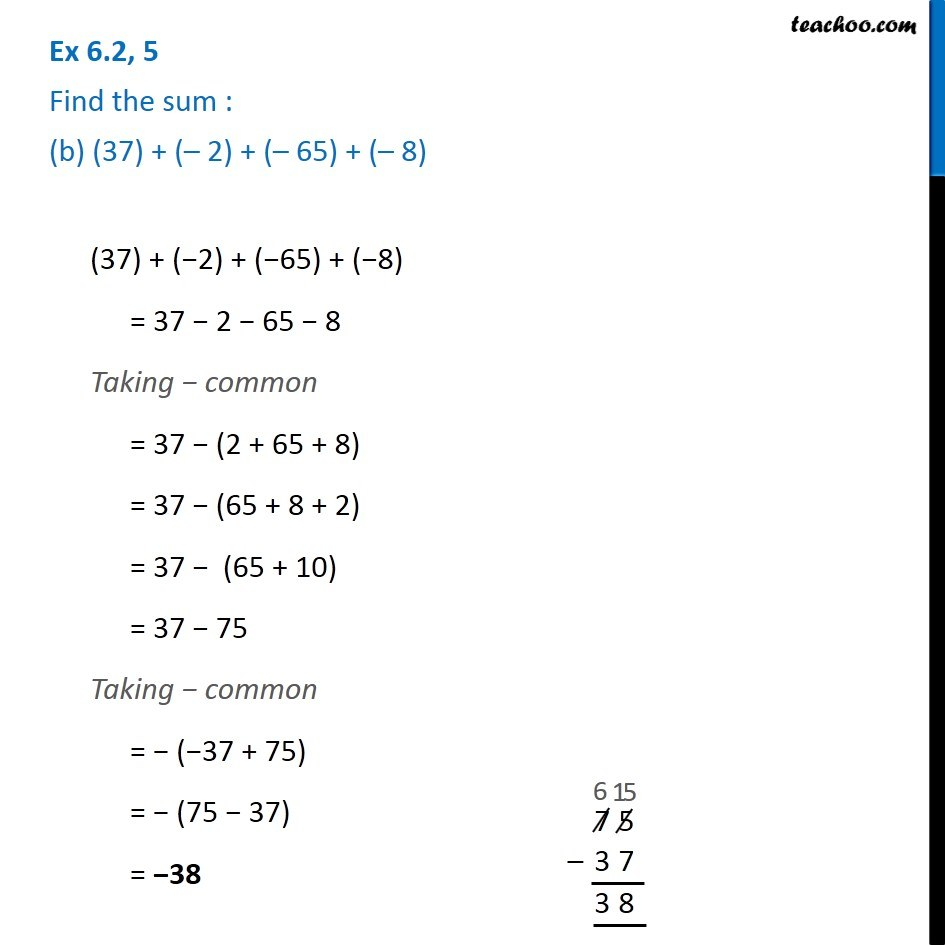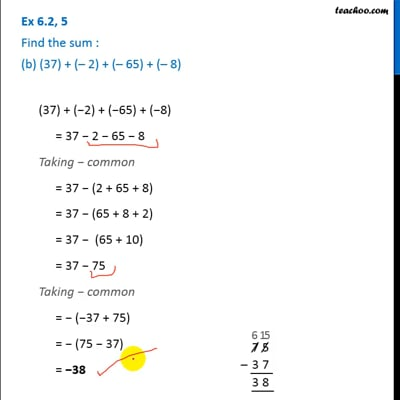Ex 6.2

Chapter 6 Class 6 Integers
Serial order wiseThis video is only available for Teachoo black users

### Transcript

Ex 6.2, 5 Find the sum : (b) (37) + ( 2) + ( 65) + ( 8) (37) + ( 2) + ( 65) + ( 8) = 37 2 65 8 Taking common = 37 (2 + 65 + 8) = 37 (65 + 8 + 2) = 37 (65 + 10) = 37 75 Taking common = ( 37 + 75) = (75 37) = 38